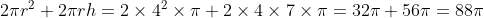## Example Questions

### Example Question #1 : Cylinders

An upright cylinder with a height of 30 and a radius of 5 is in a big tub being filled with oil. If only the top 10% of the cylinder is visible, what is the surface area of the submerged cylinder?

345π

300π

295π

325π

270π

295π

Explanation:

The height of the submerged part of the cylinder is 27cm. 2πrh + πr2 is equal to 270π + 25π = 295π

### Example Question #1 : How To Find Surface Area

A right circular cylinder has a height of 41 in. and a lateral area (excluding top and bottom) 512.5π in2. What is the area of its bases?

312.5 in2

156.25 in2

39.0625π in2

78.125π in2

78.125π in2

Explanation:

The lateral area (not including its bases) is equal to the circumference of the base times the height of the cylinder. Think of it like a label that is wrapped around a soup can. Therefore, we can write this area as:

A = h * π * d or A = h * π * 2r = 2πrh

Now, substituting in our values, we get:

512.5π = 2 * 41*rπ; 512.5π = 82rπ

Solve for r by dividing both sides by 82π:

6.25 = r

From here, we can calculate the area of a base:

A = 6.252π = 39.0625π

NOTE: The question asks for the area of the bases. Therefore, the answer is 2 * 39.0625π or 78.125π in2.

### Example Question #1 : How To Find The Surface Area Of A Cylinder

The diameter of the lid of a right cylindrical soup can is 5 in. If the can is 12 inches tall and the label costs $0.00125 per square inch to print, what is the cost to produce a label for a can? (Round to the nearest cent.) Possible Answers:$0.08

$1.18$0.29

$0.16$0.24

$0.24 Explanation: The general mechanics of this problem are simple. The lateral area of a right cylinder (excluding its top and bottom) is equal to the circumference of the top times the height of the cylinder. Therefore, the area of this can's surface is: 5π * 12 or 60π. If the cost per square inch is$0.00125, a single label will cost 0.00125 * 60π or $0.075π or approximately$0.24.

### Example Question #1 : How To Find The Surface Area Of A Cylinder

Aluminum is sold to a soup manufacturer at a rate of $0.0015 per square inch. The cans are made so that the ends perfectly fit on the cylindrical body of the can. It costs$0.00125 to attach the ends to the can. The outer label (not covering the top / bottom) costs $0.0001 per in2 to print and stick to the can. The label must be 2 inches longer than circumference of the can. Ignoring any potential waste, what is the manufacturing cost (to the nearest cent) for a can with a radius of 5 inches and a height of 12 inches? Possible Answers:$0.45

$0.84$0.57

$0.91$0.77

$0.84 Explanation: We have the following categories to consider: <Aluminum Cost> = (<Area of the top and bottom of the can> + <Lateral area of the can>) * 0.0015 <Label Cost> = (<Area of Label>) * 0.0001 <Attachment cost> = 2 * 0.00125 =$0.0025

The area of ends of the can are each equal to π*52 or 25π. For two ends, that is 50π.

The lateral area of the can is equal to the circumference of the top times the height, or 2 * π * r * h = 2 * 5 * 12 * π = 120π.

Therefore, the total surface area of the aluminum can is 120π + 50π = 170π.  The cost is 170π * 0.0015 = 0.255π, or approximately $0.80. The area of the label is NOT the same as the lateral area of the can. (Recall that it must be 2 inches longer than the circumference of the can.) Therefore, the area of the label is (2 + 2 * π * 5) * 12 = (2 + 10π) * 12 = 24 + 120π. Multiply this by 0.0001 to get 0.0024 + 0.012π = (approximately)$0.04.

Therefore, the total cost is approximately 0.80 + 0.04 + 0.0025 = $0.8425, or$0.84.

### Example Question #2 : How To Find Surface Area

The number of square units in the surface area of a right circular cylinder is equal to the number of cubic units in its volume. If r and h represent the length in units of the cylinder's radius and height, respectively, which of the following is equivalent to r in terms of h?

r = 2h2 + 2

r = 2h/(h – 2)

r = h/(2h – 2)

r = h2/(h + 2)

r = h2 + 2h

r = 2h/(h – 2)

Explanation:

We need to find expressions for the surface area and the volume of a cylinder. The surface area of the cylinder consists of the sum of the surface areas of the two bases plus the lateral surface area.

surface area of cylinder = surface area of bases + lateral surface area

The bases of the cylinder will be two circles with radius r. Thus, the area of each will be πr2, and their combined surface area will be 2πr2.

The lateral surface area of the cylinder is equal to the circumference of the circular base multiplied by the height. The circumferece of a circle is 2πr, and the height is h, so the lateral area is 2πrh.

surface area of cylinder = 2πr2 + 2πrh

Next, we need to find an expression for the volume. The volume of a cylinder is equal to the product of the height and the area of one of the bases. The area of the base is πr2, and the height is h, so the volume of the cylinder is πr2h.

volume = πr2h

Then, we must set the volume and surface area expressions equal to one another and solve for r in terms of h.

2πr2 + 2πrhπr2h

First, let's factor out 2πr from the left side.

2πr(h) = πr2h

We can divide both sides by π.

2r(h) = r2h

We can also divide both sides by r, because the radius cannot equal zero.

2(h) = rh

Let's now distribute the 2 on the left side.

2r + 2h = rh

Subtract 2r from both sides to get all the r's on one side.

2h = rh – 2r

rh – 2r = 2h

Factor out an r from the left side.

r(h – 2) = 2h

Divide both sides by h – 2

r = 2h/(h – 2)

The answer is r = 2h/(h – 2).

### Example Question #1 : How To Find The Surface Area Of A Cylinder

What is the surface area of a cylinder with a radius of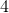and a height of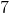?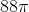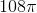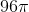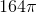Therefore the surface area of a cylinder =